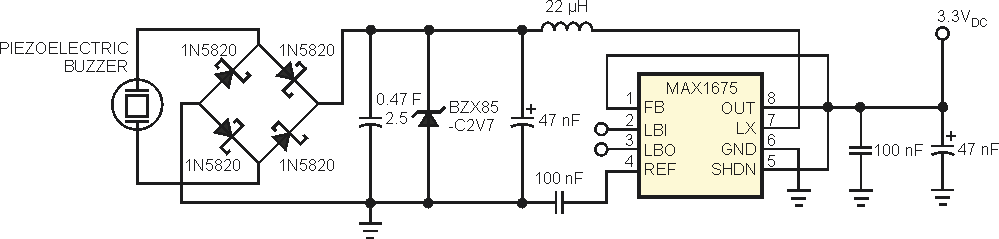# Harvest Energy Using a Piezoelectric Buzzer

## Maxim MAX1675

Energy-harvesting, or “scavenging,” systems extract energy from the ambient environment. Unfortunately, these power generators supply much less energy than do standard batteries. However, thanks to the decreasing size and low-power requirements of today’s wearable devices, it is feasible to replace batteries in some low-power systems with power generators that capture energy from the user’s environment, such as the vibration energy a user produces during walking or running. This Design Idea uses the piezoelectric effect of a standard and easy-to-find piezoelectric buzzer to turn mechanical vibrations into electrical energy. Although piezoelectric buzzers generate sound waves when you apply an ac voltage, you can use them in the opposite way: You obtain the maximum ac peak voltage that the piezoelectric buzzer generates when the vibration frequency matches the resonant frequency of the piezoelectric buzzer.

The power generator in Figure 1 is a simple circuit. The piezoelectric buzzer produces an ac voltage when it is under vibration; therefore, you must convert this voltage to a dc voltage before charging the capacitor. The four Schottky diodes form a bridge rectifier to perform this task. For a reliable and efficient operation, select Schottky diodes, such as the 1N5820 rectifier diode from ON Semiconductor, that exhibit low forward-voltage drop and low reverse leakage.Figure 1. A simple vibrational-energy-harvesting circuit using an off-the-shelf piezoelectric buzzer as the power generator turns mechanical energy into electrical energy.

Energy harvesters typically capture small amounts of energy over long periods, so harvesters usually contain an energy-storage subsystem in the form of a supercapacitor, such as the PowerStor 0.47 F, 2.5 V capacitor from Cooper Busmann. The larger the capacitor, the longer it takes to charge it. On the other hand, a larger capacitor provides power for a longer time for the same load. Because a supercapacitor often has a much lower voltage than standard electrolytic capacitors, you must connect a zener diode, such as the BZX85-C2V7, to prevent the voltage across the supercapacitor from increasing beyond its maximum voltage rating. As soon as you apply a load, the supercapacitor starts discharging, and the voltage across the supercapacitor starts dropping. To guarantee a fixed voltage at the output, you must use a dc/dc-voltage-converter IC, such as the MAX1675 from Maxim as a step-up converter working at 3.3 V.

As an additional benefit, if the supercapacitor’s voltage drops below the required voltage of operation, the circuit continues to provide regulated output voltage as long as the supercapacitor voltage does not drop below the lower limit of the dc/dc converter. This limit is 0.7 V for the MAX1675.

EDN